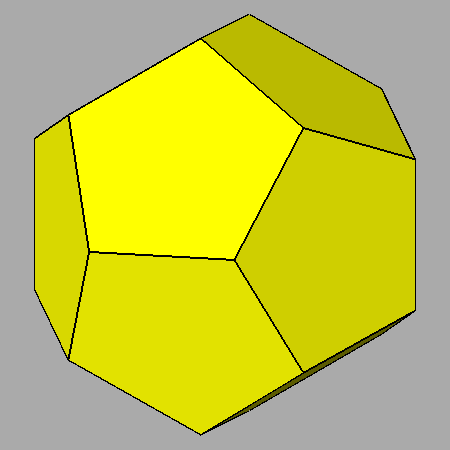Acronym ... Name icosahedrally truncated rhombic triacontahedron,chamfered dodecahedron,Goldberg polyhedron GP(2,0)©© Vertex figure [5,H2], [h3] General of army (is itself convex) Colonel of regiment (is itself locally convex) Confer rhote   doe ExternallinksThe non-regular hexahedra {(h,H,H)2} have vertex angles h = arccos(-1/sqrt(5)) = 116.565051° resp. H = arccos(-sqrt[(5-sqrt(5))/10]) = 121.717474°.

Icosahedral truncation applies to the icosahedral vertices (vertex inscribed ike) only. Wrt. the rhote this produces new pentagon faces there. These are then face planes of a cutting doe. – The above transition shows a dynamical mutual scaling of doe and rhote. The chamfered dodecahedron then is the instance, where all edges happen to have the same length.

Chamfering (or edge-only beveling – here being applied to the doe) flatens the former edges into new (non-regular hexagonal) faces.

There is a deeper, terminal chamfering of the doe too, which then reduces the original faces to nothing. Then the hexagons will become rhombs and the total figure becomes the rhote. – When considering the below provided tegum sum Dynkin symbol, it becomes obvious that this figure also can be seen as a Stott expansion of the rhote.

Incidence matrix according to Dynkin symbol

ax5oo3oc&#zx   → height = 0
a = 1+sqrt[(10-2 sqrt(5))/5] = 2.051462
c = 2/sqrt(3) = 1.154701
(tegum sum of a-doe and (x,c)-srid)

o.5o.3o.     | 20  * |  3  0 |  3  0  [h3]
.o5.o3.o     |  * 60 |  1  2 |  2  1  [5,H2]
-------------+-------+-------+------
oo5oo3oo&#x  |  1  1 | 60  * |  2  0
.x .. ..     |  0  2 |  * 60 |  1  1
-------------+-------+-------+------
ax .. oc&#zx |  2  4 |  4  2 | 30  *  {(h,H,H)2}
.x5.o ..     |  0  5 |  0  5 |  * 12  {5}